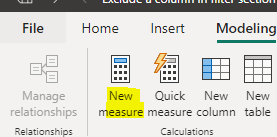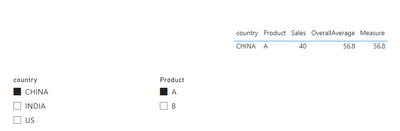cancel
Showing results for
Did you mean:New Member

## Exclude a column in filter section

Hello, I been working in a table where I need to exclude a column from alicer selection and other columns should work as per slection in slicer.

TABLE

 country Product Sales INDIA A 50 CHINA A 40 US A 70 INDIA B 56 CHINA B 85 US B 40

Expected Outcome

 Country Product OverallAverage Sales CHINA A 56.8 40

In the outcome Product will be Slicer, the Slicer slection should impact only the colums Country, Product, Sales, Where the Overall Average column should remain constance irrespective of filter selection, (Overall Average is a average of sales irrexpective of procut)

Is there a way toget this done

Thanks

1 ACCEPTED SOLUTIONCommunity Support

Hi @gowtham1991 ,

According to your description, here are my steps you can follow as a solution.

(1) My test data is the same as yours.

(2) We can create a calculated column.``OverallAverage = AVERAGE('Table'[Sales])``

Or we can create a measure.``Measure = AVERAGEX(FILTER(ALL('Table'),'Table'[Sales]),CALCULATE(SUM('Table'[Sales])))``

(3) Then the result is as follows.If the above one can't help you get the desired result, please provide some sample data in your tables (exclude sensitive data) with Text format and your expected result with backend logic and special examples. It is better if you can share a simplified pbix file. Thank you.

Best Regards,

Neeko Tang

If this post  helps, then please consider Accept it as the solution  to help the other members find it more quickly.

2 REPLIES 2Super User

Hi,

These measures work

``S = SUM(Data[Sales])``
``Measure = AVERAGEX(ALL(Data),[S])``

Hope this helps.Regards,
Ashish Mathur
http://www.ashishmathur.comCommunity Support

Hi @gowtham1991 ,

According to your description, here are my steps you can follow as a solution.

(1) My test data is the same as yours.

(2) We can create a calculated column.``OverallAverage = AVERAGE('Table'[Sales])``

Or we can create a measure.``Measure = AVERAGEX(FILTER(ALL('Table'),'Table'[Sales]),CALCULATE(SUM('Table'[Sales])))``

(3) Then the result is as follows.If the above one can't help you get the desired result, please provide some sample data in your tables (exclude sensitive data) with Text format and your expected result with backend logic and special examples. It is better if you can share a simplified pbix file. Thank you.

Best Regards,

Neeko Tang

If this post  helps, then please consider Accept it as the solution  to help the other members find it more quickly.